Скачать презентацию Fast Approximately Optimal Solutions for Single and Dynamic

fc552126bc0e238ae3bfe2413556659c.ppt

• Количество слайдов: 20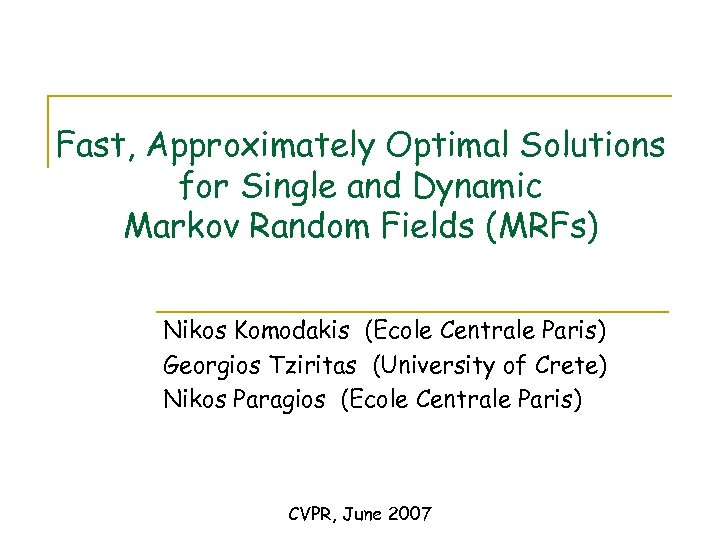Fast, Approximately Optimal Solutions for Single and Dynamic Markov Random Fields (MRFs) Nikos Komodakis (Ecole Centrale Paris) Georgios Tziritas (University of Crete) Nikos Paragios (Ecole Centrale Paris) CVPR, June 2007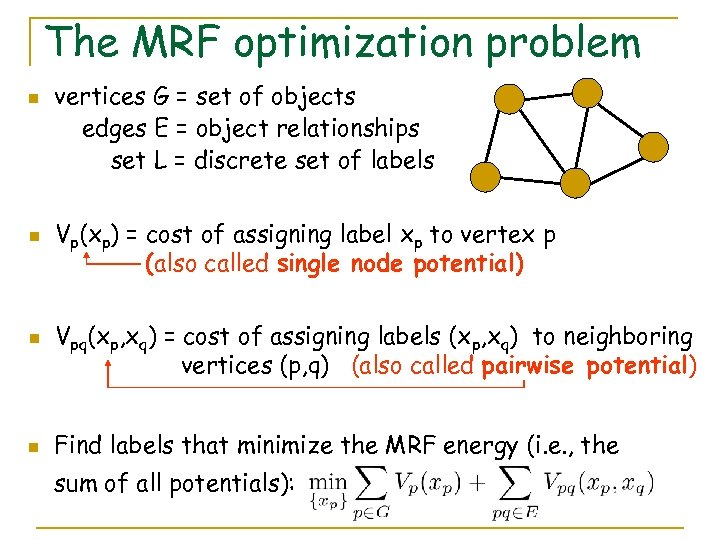The MRF optimization problem n n vertices G = set of objects edges E = object relationships set L = discrete set of labels Vp(xp) = cost of assigning label xp to vertex p (also called single node potential) Vpq(xp, xq) = cost of assigning labels (xp, xq) to neighboring vertices (p, q) (also called pairwise potential) Find labels that minimize the MRF energy (i. e. , the sum of all potentials):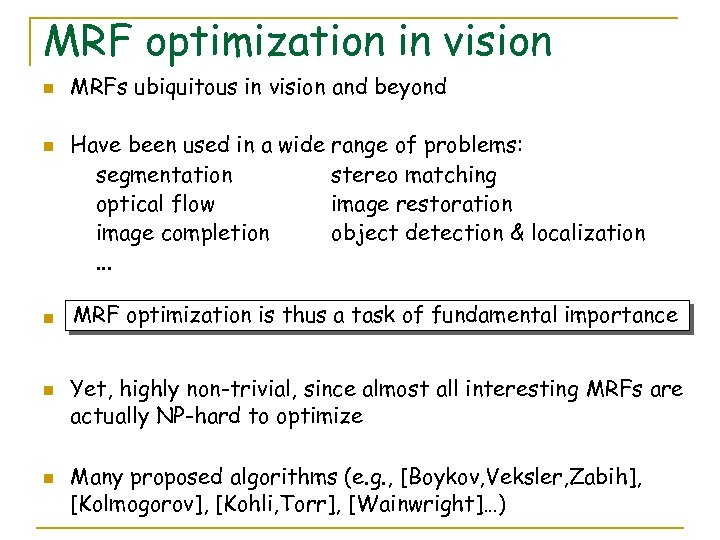MRF optimization in vision n n MRFs ubiquitous in vision and beyond Have been used in a wide range of problems: segmentation stereo matching optical flow image restoration image completion object detection & localization. . . MRF optimization is thus a task of fundamental importance Yet, highly non-trivial, since almost all interesting MRFs are actually NP-hard to optimize Many proposed algorithms (e. g. , [Boykov, Veksler, Zabih], [Kolmogorov], [Kohli, Torr], [Wainwright]…)MRF hardness local optimum global optimum approximation exact global optimum MRF pairwise potential linear metric arbitrary n n Move left in the horizontal axis, and remain low in the vertical axis (i. e. , still be able to provide approximately optimal solutions) But we want to be able to do that efficiently, i. e. fast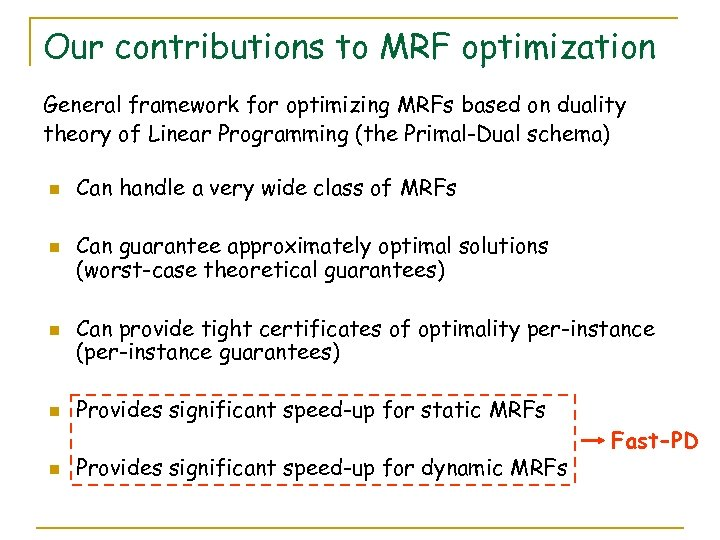Our contributions to MRF optimization General framework for optimizing MRFs based on duality theory of Linear Programming (the Primal-Dual schema) n n n Can handle a very wide class of MRFs Can guarantee approximately optimal solutions (worst-case theoretical guarantees) Can provide tight certificates of optimality per-instance (per-instance guarantees) Provides significant speed-up for static MRFs Provides significant speed-up for dynamic MRFs Fast-PD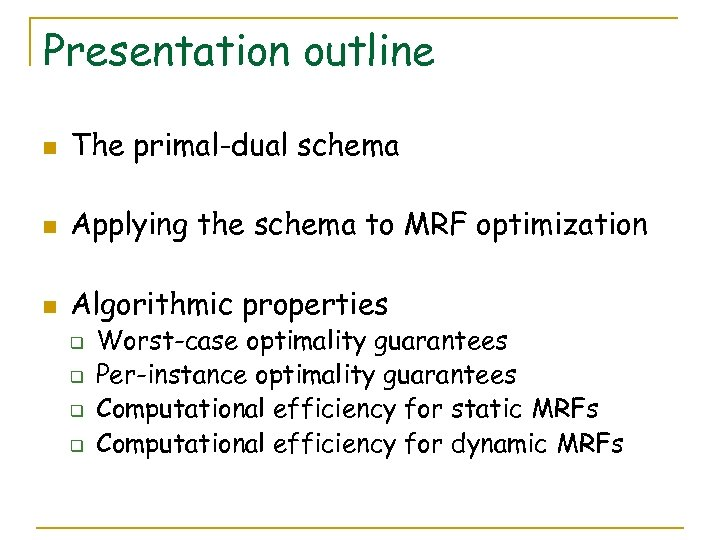Presentation outline n The primal-dual schema n Applying the schema to MRF optimization n Algorithmic properties q q Worst-case optimality guarantees Per-instance optimality guarantees Computational efficiency for static MRFs Computational efficiency for dynamic MRFs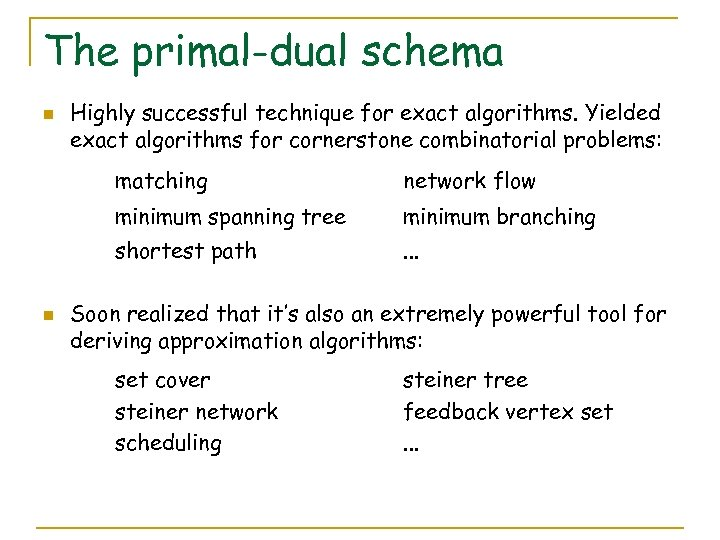The primal-dual schema n Highly successful technique for exact algorithms. Yielded exact algorithms for cornerstone combinatorial problems: matching minimum spanning tree minimum branching shortest path n network flow. . . Soon realized that it’s also an extremely powerful tool for deriving approximation algorithms: set cover steiner network scheduling steiner tree feedback vertex set. . .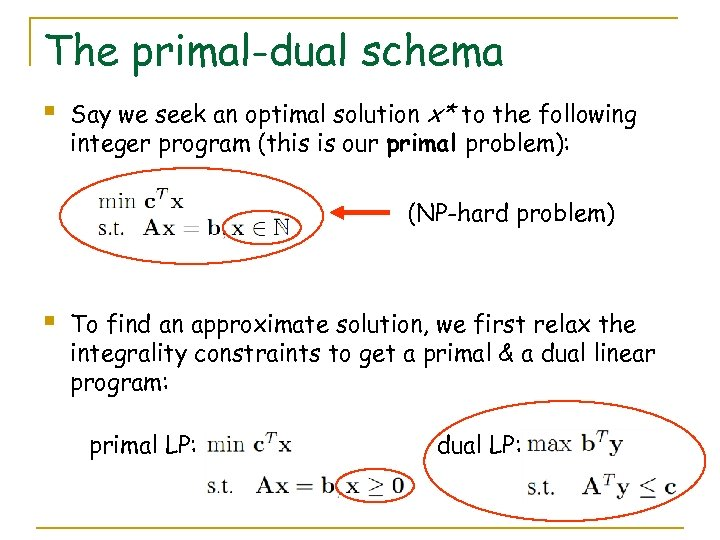The primal-dual schema § Say we seek an optimal solution x* to the following integer program (this is our primal problem): (NP-hard problem) § To find an approximate solution, we first relax the integrality constraints to get a primal & a dual linear program: primal LP: dual LP: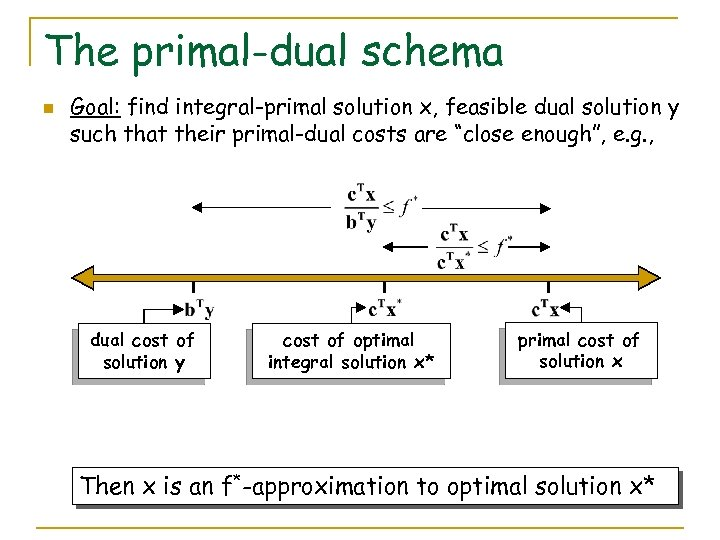The primal-dual schema n Goal: find integral-primal solution x, feasible dual solution y such that their primal-dual costs are “close enough”, e. g. , dual cost of solution y cost of optimal integral solution x* primal cost of solution x Then x is an f*-approximation to optimal solution x*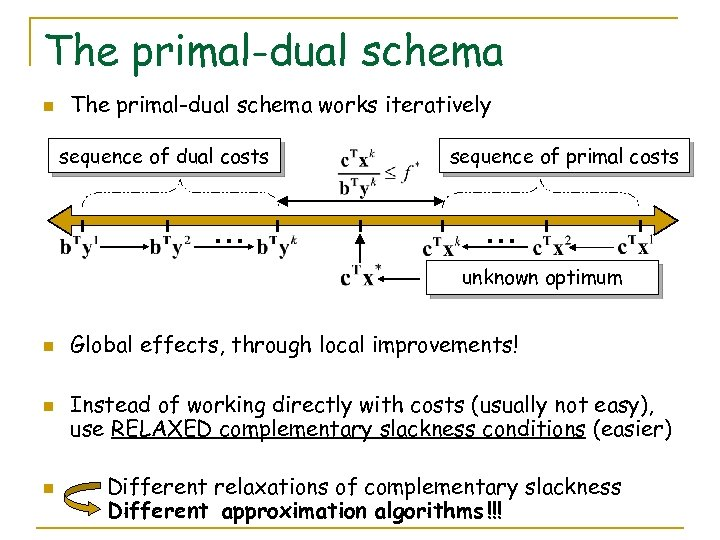The primal-dual schema n The primal-dual schema works iteratively sequence of dual costs … sequence of primal costs … unknown optimum n n n Global effects, through local improvements! Instead of working directly with costs (usually not easy), use RELAXED complementary slackness conditions (easier) Different relaxations of complementary slackness Different approximation algorithms !!!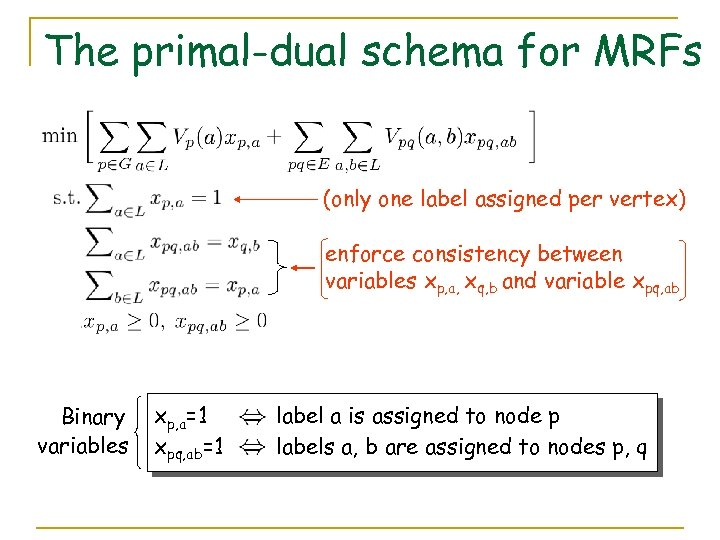The primal-dual schema for MRFs (only one label assigned per vertex) enforce consistency between variables xp, a, xq, b and variable xpq, ab Binary variables xp, a=1 xpq, ab=1 label a is assigned to node p labels a, b are assigned to nodes p, q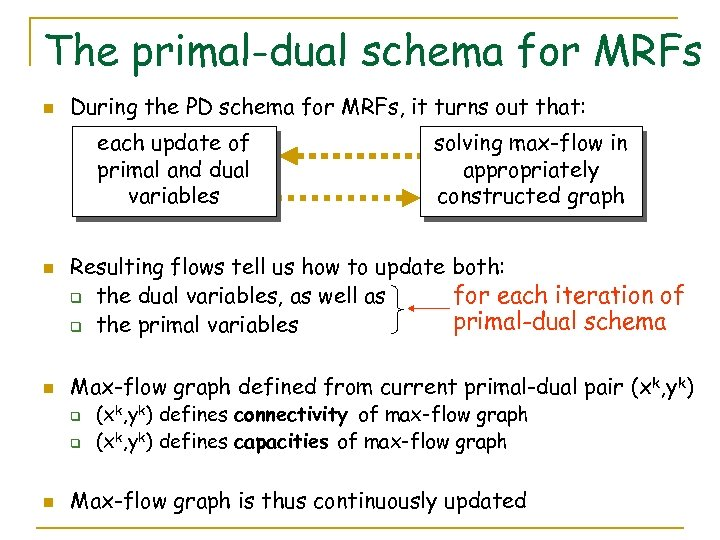The primal-dual schema for MRFs n During the PD schema for MRFs, it turns out that: each update of primal and dual variables n n Resulting flows tell us how to update both: for each iteration of q the dual variables, as well as primal-dual schema q the primal variables Max-flow graph defined from current primal-dual pair (xk, yk) q q n solving max-flow in appropriately constructed graph (xk, yk) defines connectivity of max-flow graph (xk, yk) defines capacities of max-flow graph Max-flow graph is thus continuously updated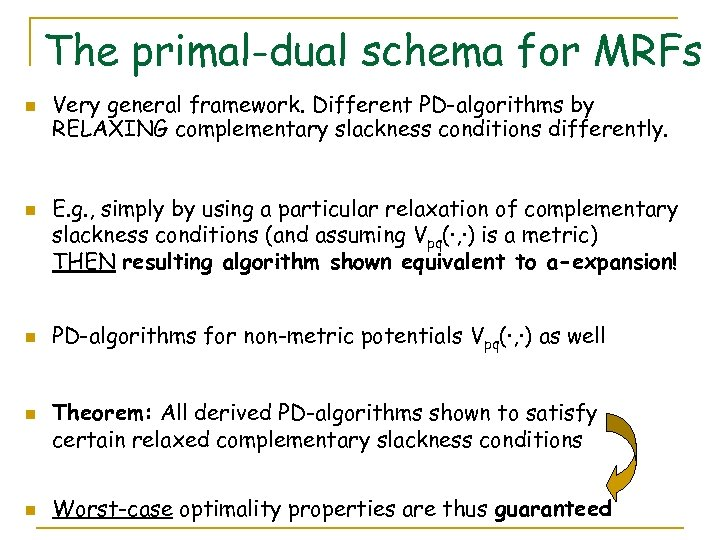The primal-dual schema for MRFs n n n Very general framework. Different PD-algorithms by RELAXING complementary slackness conditions differently. E. g. , simply by using a particular relaxation of complementary slackness conditions (and assuming Vpq(·, ·) is a metric) THEN resulting algorithm shown equivalent to a-expansion! PD-algorithms for non-metric potentials Vpq(·, ·) as well Theorem: All derived PD-algorithms shown to satisfy certain relaxed complementary slackness conditions Worst-case optimality properties are thus guaranteed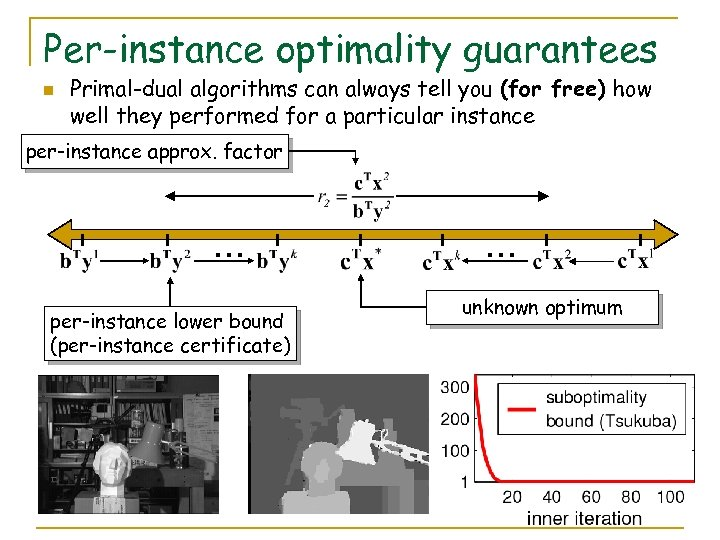Per-instance optimality guarantees n Primal-dual algorithms can always tell you (for free) how well they performed for a particular instance per-instance approx. factor … per-instance lower bound (per-instance certificate) … unknown optimum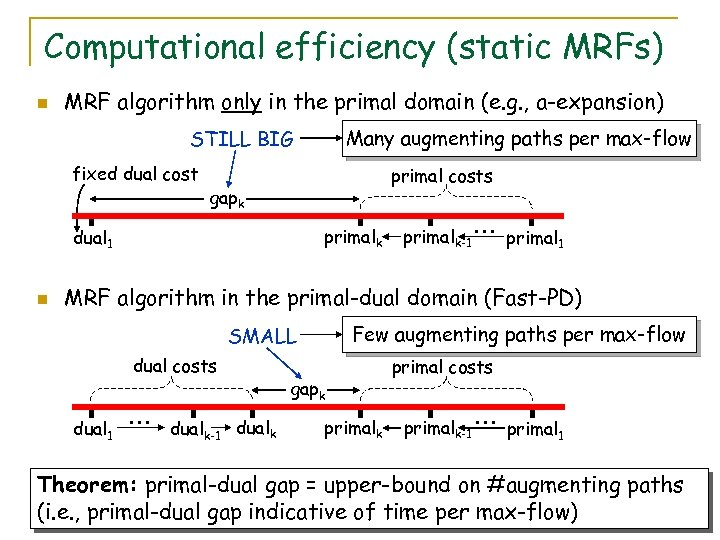Computational efficiency (static MRFs) n MRF algorithm only in the primal domain (e. g. , a-expansion) Many augmenting paths per max-flow STILL BIG fixed dual cost primal costs gapk primalk dual 1 n primalk-1 … primal 1 MRF algorithm in the primal-dual domain (Fast-PD) Few augmenting paths per max-flow SMALL dual costs dual 1 … dualk-1 dualk gapk primal costs primalk-1 … primal 1 Theorem: primal-dual gap = upper-bound on #augmenting paths (i. e. , primal-dual gap indicative of time per max-flow)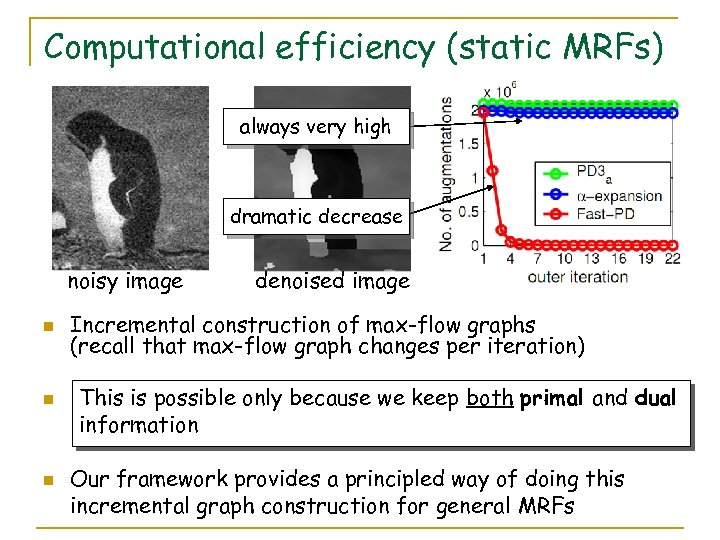Computational efficiency (static MRFs) always very high dramatic decrease noisy image n n n denoised image Incremental construction of max-flow graphs (recall that max-flow graph changes per iteration) This is possible only because we keep both primal and dual information Our framework provides a principled way of doing this incremental graph construction for general MRFs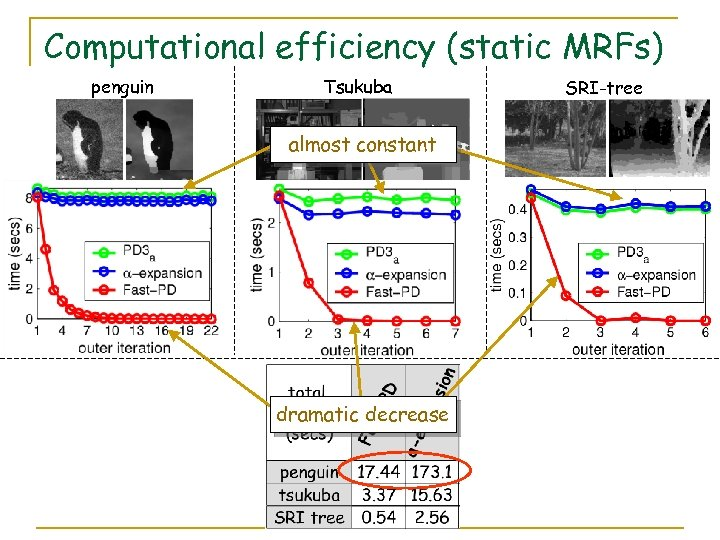Computational efficiency (static MRFs) penguin Tsukuba almost constant dramatic decrease SRI-tree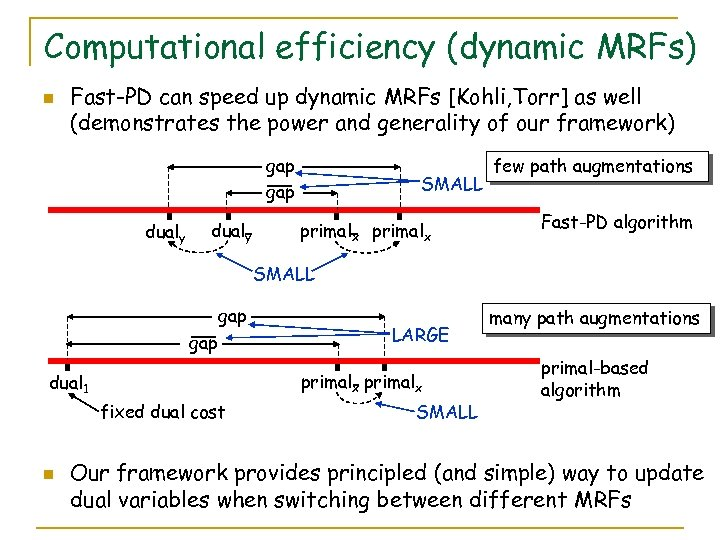Computational efficiency (dynamic MRFs) n Fast-PD can speed up dynamic MRFs [Kohli, Torr] as well (demonstrates the power and generality of our framework) gap dualy SMALL primalx few path augmentations Fast-PD algorithm SMALL gap primalx dual 1 fixed dual cost n LARGE SMALL many path augmentations primal-based algorithm Our framework provides principled (and simple) way to update dual variables when switching between different MRFs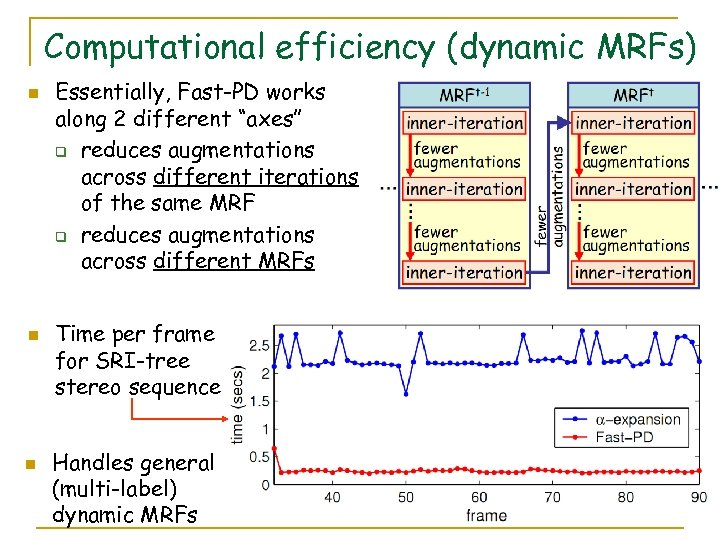Computational efficiency (dynamic MRFs) n n n Essentially, Fast-PD works along 2 different “axes” q reduces augmentations across different iterations of the same MRF q reduces augmentations across different MRFs Time per frame for SRI-tree stereo sequence Handles general (multi-label) dynamic MRFs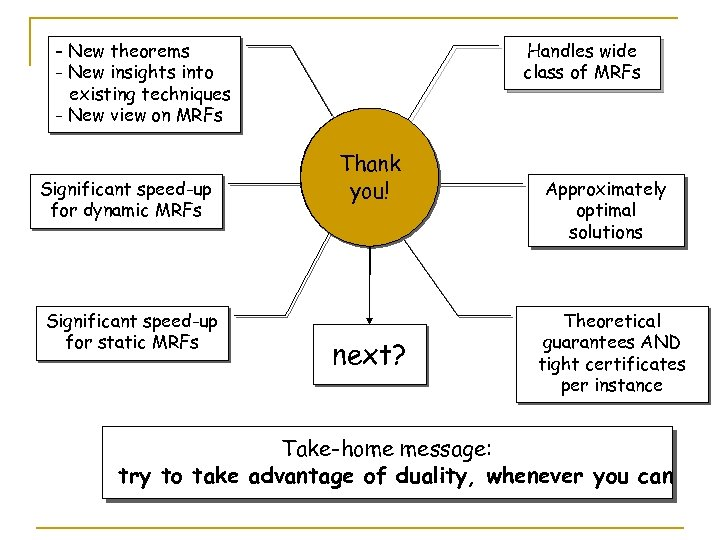- New theorems - New insights into existing techniques - New view on MRFs Significant speed-up for dynamic MRFs Significant speed-up for static MRFs Handles wide class of MRFs primal-dual Thank framework you! next? Approximately optimal solutions Theoretical guarantees AND tight certificates per instance Take-home message: try to take advantage of duality, whenever you can